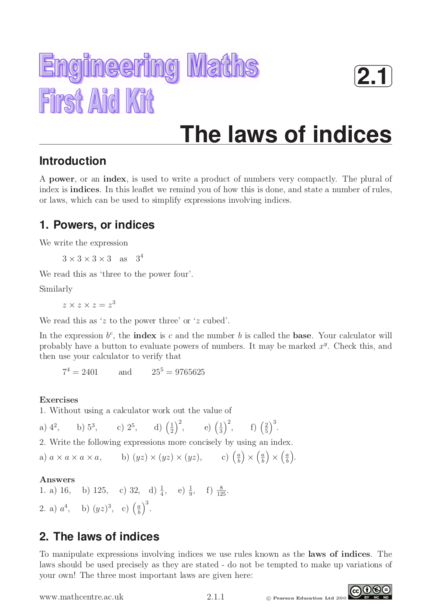# Law of IndicesContributed by:This includes the Laws of indices that provide us with rules for simplifying calculations or expressions involving powers of the same base.
1. 
2.1
The laws of indices
A power, or an index, is used to write a product of numbers very compactly. The plural of
index is indices. In this leaflet we remind you of how this is done, and state a number of rules,
or laws, which can be used to simplify expressions involving indices.
1. Powers, or indices
We write the expression
3 × 3 × 3 × 3 as 34
We read this as ‘three to the power four’.
z × z × z = z3
We read this as ‘z to the power three’ or ‘z cubed’.
In the expression bc , the index is c and the number b is called the base. Your calculator will
probably have a button to evaluate powers of numbers. It may be marked xy . Check this, and
then use your calculator to verify that
74 = 2401 and 255 = 9765625
1. Without using a calculator work out the value of
 2  2  3
1 1 2
a) 42 , b) 53 , c) 25 , d) 2
, e) 3
, f) 5
.
2. Write the following expressions more concisely by using an index.
     
a a a
a) a × a × a × a, b) (yz) × (yz) × (yz), c) b
× b
× b
.
1. a) 16, b) 125, c) 32, d) 41 , e) 91 , f) 8
125
.
 3
a
2. a) a4 , b) (yz)3 , c) b
.
2. The laws of indices
To manipulate expressions involving indices we use rules known as the laws of indices. The
laws should be used precisely as they are stated - do not be tempted to make up variations of
your own! The three most important laws are given here:
www.mathcentre.ac.uk 2.1.1 c Pearson Education Ltd 2000
2. First law
am × an = am+n
When expressions with the same base are multiplied, the indices are added.
We can write
76 × 74 = 76+4 = 710
You could verify this by evaluating both sides separately.
z 4 × z 3 = z 4+3 = z 7
Second Law
am
= am−n
an
When expressions with the same base are divided, the indices are subtracted.
We can write
85 z7
= 85−3 = 82 and similarly = z 7−4 = z 3
8 3 z 4
Third law
(am )n = amn
Note that m and n have been multiplied to yield the new index mn.
(64 )2 = 64×2 = 68 and (ex )y = exy
It will also be useful to note the following important results:
a0 = 1, a1 = a
1. In each case choose an appropriate law to simplify the expression:
y7 x8
a) 53 × 513 , b) 813 ÷ 85 , c) x6 × x5 , d) (a3 )4 , e) y3
, f) x7
.
2. Use one of the laws to simplify, if possible, a6 × b5 .
1. a) 516 , b) 88 , c) x11 , d) a12 , e) y 4 , f) x1 = x.
2. This cannot be simplified because the bases are not the same.
www.mathcentre.ac.uk 2.1.2 c Pearson Education Ltd 2000